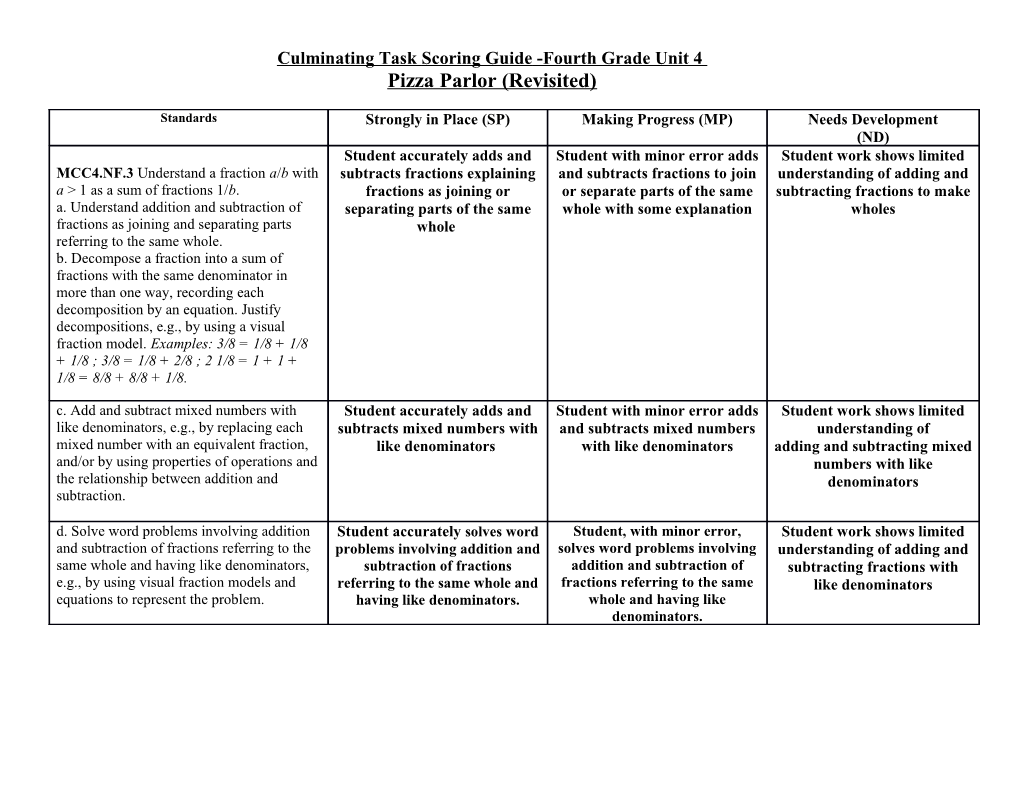Pizza Parlor (Revisited)

Standards / Strongly in Place (SP) / Making Progress (MP) / Needs Development
(ND)
MCC4.NF.3 Understand a fraction a/b with a > 1 as a sum of fractions 1/b.
a. Understand addition and subtraction of fractions as joining and separating parts referring to the same whole.
b. Decompose a fraction into a sum of fractions with the same denominator in more than one way, recording each decomposition by an equation. Justify decompositions, e.g., by using a visual fraction model. Examples: 3/8 = 1/8 + 1/8 + 1/8 ; 3/8 = 1/8 + 2/8 ; 2 1/8 = 1 + 1 + 1/8 = 8/8 + 8/8 + 1/8. / Student accurately adds and subtracts fractions explaining fractions as joining or separating parts of the same whole / Student with minor error adds and subtracts fractions to join or separate parts of the same whole with some explanation / Student work shows limited understanding of adding and subtracting fractions to make wholes
c. Add and subtract mixed numbers with like denominators, e.g., by replacing each mixed number with an equivalent fraction, and/or by using properties of operations and the relationship between addition and subtraction. / Student accurately adds and subtracts mixed numbers with like denominators / Student with minor error adds and subtracts mixed numbers with like denominators / Student work shows limited understanding of
adding and subtracting mixed numbers with like denominators
d. Solve word problems involving addition and subtraction of fractions referring to the same whole and having like denominators, e.g., by using visual fraction models and equations to represent the problem. / Student accurately solves word problems involving addition and subtraction of fractions referring to the same whole and having like denominators. / Student, with minor error, solves word problems involving addition and subtraction of fractions referring to the same whole and having like denominators. / Student work shows limited understanding of adding and subtracting fractions with like denominators
SMP / Strongly in Place / Satisfactory / Making Progress/ Developing / Area of Concern
Make sense of problems and persevere in solving them. / The student explained the problem and showed perseverance by identifying the purpose of the problem and selected and applied an appropriate problem solving strategy that lead to a thorough and accurate solution. / The student explained the problem and showed perseverance when identifying the purpose of the problem, and selected an applied and appropriate problem solving strategy that lead to a generally complete and accurate solution. / The student explained the problem and showed some perseverance in identifying the purpose of the problem, and selected and applied an appropriate problem solving strategy that lead to a partially complete and/or partially accurate solution. / The student was unable to explain the problem and showed minimal perseverance when identifying the purpose of the problem.
Attends to precision / The student was precise by clearly describing their actions and strategies, while showing understanding and using above-grade-level appropriate vocabulary in their process of finding solutions. / The student was precise by clearly describing their actions and strategies, while showing understanding and using grade-level appropriate vocabulary in their process of finding solutions. / The student was precise by clearly describing their actions and strategies, while showing understanding and using appropriate vocabulary in their process of finding solutions. / The student was unclear in their thinking and was unable to communicate mathematically.
Reasoning and Explaining / The student expressed and justified their opinion both quantitatively and abstractly using a variety of numbers, pictures, charts and words. / The student expressed and justified their opinion both quantitatively and abstractly using numbers, pictures, charts and/or words. / The student expressed or justified their opinion either quantitatively OR abstractly using numbers, pictures, charts OR words. / The student was unable to express or justify their opinion quantitatively or abstractly using numbers, pictures, charts or words.
Models and use of tools / The student selected multiple efficient tools and correctly represented the tools to reason and justify their response. In addition this students was able to explain why their tool/ model was efficient / The student selected an efficient tool and/or drew a correct representation of the efficient tool used to reason and justify their response. / The student selected appropriate tools or drew a correct representation of the tools used to reason and justify their response. / The student was unable to select an appropriate tool, draw a representation to reason or justify their thinking.
Seeing structure and generalizing / The student identified various patterns and structures in the number system and noticed connections to multiple areas of mathematics and real-life. / The student identified patterns or structures in the number system and noticed connections to other areas of mathematics and real-life. / The student identified a pattern or structure in the number system and noticed connections to other areas of mathematics or real-life. / The student was unable to identify patterns, structures or connect to other areas of mathematics and/or real-life.